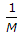# Civil Engineering - Theory of Structures

Exercise : Theory of Structures - Section 2
41.
A road of uniform cross-section A and length L is deformed by δ, when subjected to a normal force P. The Young's Modulus E of the material, is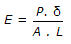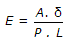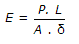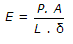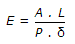Explanation:
No answer description is available. Let's discuss.

42.
The S.F. diagram of a loaded beam shown in the given figure is that of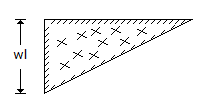a simply supported beam with isolated central load
a simply supported beam with uniformly distributed load
a cantilever with an isolated load at the free end
a cantilever with a uniformly distributed load.
Explanation:
No answer description is available. Let's discuss.

43.
An isolated load W is acting at a distance a from the left hand support, of a three hinged arch of span 2l and rise h hinged at the crown, the horizontal reaction at the support, is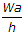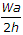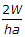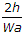Explanation:
No answer description is available. Let's discuss.

44.
The ratio of lateral strain to axial strain of a homogeneous material, is known
Yield ratio
Hooke's ratio
Poisson's ratio
Plastic ratio.
width b